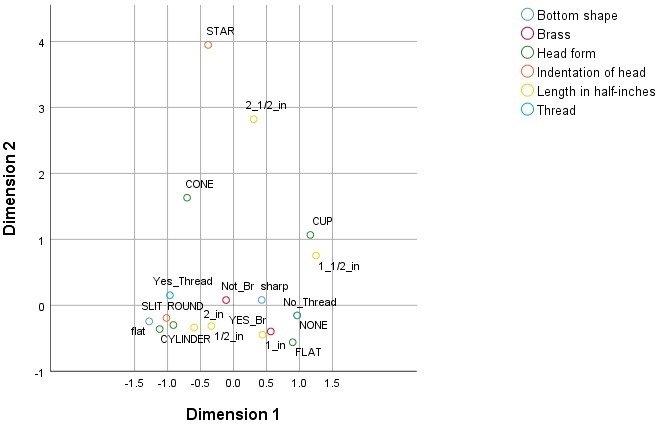## With IBM SPSS Categories software, you can:

• Visualize and explore complex categorical and numeric data as well as high-dimensional data
• Understand information in large two-way and multiway tables
• Use biplots, triplots and perceptual maps to uncover relationships in your data

## Present your results clearly using perceptual maps

Use dimension reduction techniques to go beyond unwieldy tables to clearly see relationships in your data using revealing perceptual maps and biplots. Summary charts display similar variables or categories, providing you with insight into relationships among more than two variables.

## Unleash the full potential of your data through optimal scaling and dimension reduction techniques

Correspondence analysis (CORRESPONDENCE): Describe the relationships between two nominal variables in a low-dimensional space while simultaneously describing the relationships between categories for each variable.

Categorical regression (CATREG): Predict the values of a categorical dependent variable from a combination of categorical independent variables. Optimal scaling techniques quantify the variables in such a way that the Multiple R is maximized. Regularization methods improve prediction accuracy by stabilizing the parameter estimates.

Multiple correspondence analysis (MULTIPLE CORRESPONDENCE):Analyze a categorical multivariate data matrix for two or more nominal variables.

CATPCA: Use alternating least squares to generalize principal components analysis to accommodate variables of mixed measurement levels. Specify a transformation type of nominal, ordinal or numeric on a variable-by-variable basis.

Nonlinear canonical correlation (OVERALS):Use alternating least squares to generalize canonical correlation analysis. It allows more than one set of variables to be compared to one another on the same graph.

Proximity scaling (PROXSCAL): Takes a matrix of similarity and dissimilarity distances between observations in a highdimensional space and assigns them to a position in a lowdimensional space so you can gain spatial understanding of how objects relate.

Preference scaling (PREFSCAL): Set up the preference scaling procedure (PREFSCAL) in syntax to perform multidimensional unfolding on two sets of objects to find a map that represents the relationships between these two sets of objects as distances between two sets of points.

## System Screen

The distance from an object to the origin reflects variation from the “average” response pattern. This average response pattern corresponds to the most frequent category for each variable. Objects with many characteristics corresponding to the most frequent categories lie near the origin. In contrast, objects with unique characteristics are located far from the origin.